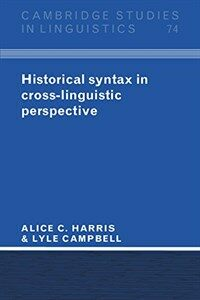> Detail View

# Detail View## Historical syntax in cross-linguistic perspective

Material type
단행본
Personal Author
Harris, Alice C. Campbell, Lyle.
Title Statement
Historical syntax in cross-linguistic perspective / Alice C. Harris, Lyle Campbell.
Publication, Distribution, etc
Cambridge ;   New York :   Cambridge University Press,   1995.
Physical Medium
xvii, 488 p. : ill. ; 24 cm.
Series Statement
Cambridge studies in linguistics ;74
ISBN
0521472946 (hardback) 0521478812 (pbk.)
Bibliography, Etc. Note
Includes bibliographical references (p. 436-477) and indexes.
Subject Added Entry-Topical Term
Grammar, Comparative and general -- Syntax. Historical linguistics.
 000 01111camuu2200325 a 4500 001 000045726817 005 20121102094741 008 121101s1995 enka b 001 0 eng 010 ▼a 94015013 020 ▼a 0521472946 (hardback) 020 ▼a 0521478812 (pbk.) 035 ▼a (KERIS)REF000014724864 040 ▼a DLC ▼c DLC ▼d DLC ▼d 211009 041 0 ▼a eng ▼a mul 050 0 0 ▼a P291 ▼b .H25 1995 082 0 0 ▼a 415 ▼2 23 084 ▼a 415 ▼2 DDCK 090 ▼a 415 ▼b H313h 100 1 ▼a Harris, Alice C. 245 1 0 ▼a Historical syntax in cross-linguistic perspective / ▼c Alice C. Harris, Lyle Campbell. 260 ▼a Cambridge ; ▼a New York : ▼b Cambridge University Press, ▼c 1995. 300 ▼a xvii, 488 p. : ▼b ill. ; ▼c 24 cm. 490 1 ▼a Cambridge studies in linguistics ; ▼v 74 504 ▼a Includes bibliographical references (p. 436-477) and indexes. 650 0 ▼a Grammar, Comparative and general ▼x Syntax. 650 0 ▼a Historical linguistics. 700 1 ▼a Campbell, Lyle. 830 0 ▼a Cambridge studies in linguistics ; ▼v 74. 945 ▼a KLPA

### Holdings Information

No. Location Call Number Accession No. Availability Due Date Make a Reservation Service
No. 1 Location Call Number 415 H313h Accession No. 111678942 Availability Available Due Date Make a Reservation Service

### Contents information

```
CONTENTS
Preface = xiii
List of abbreviations = xv
1 Introduction = 1
1.1 Goals of a theory of diachronic syntax = 1
1.2 Orientation to the organization and contents of the book = 2
1.3 On the relation to synchronic syntax = 4
1.4 On prediction = 5
1.5 On explanation = 7
1.6 Characteristics of a theory of syntactic change = 7
1.7 The method employed = 8
1.8 The syntactic framework = 9
1.9 The evidence = 10
2.The history of historical syntax: major themes = 14
2.1 Introduction = 14
2.2 Foundations of the diachronic study of syntax = 15
2.3 Historical syntax since 1957 = 34
2.4 Conclusion = 46
3 Overview of a theory of syntactic change = 48
3.1 Basic assumptions about language change = 48
3.2 Outline of a theory of syntactic change = 50
3.3 On mechanisms of syntactic change = 50
3.4 On universally available syntactic constructions = 54
3.5 On universal principles = 57
3.6 The role of operations in an account of syntactic change = 57
3.7 Why this theory? = 59
4 Reanalysis = 61
4.1 Introduction = 61
4.2 Examples of reanalysis = 65
4.3 Preludes to reanalysis = 70
4.4 Reanalysis and actualization = 77
4.5 Some types of change that may be effected by reanalysis = 89
4.6 Summary = 93
Appendix: preverbs in Kartvelian = 94
5 Extension = 97
5.1 Introduction = 97
5.2 Some examples of extension = 98
5.3 Towards a constraint on extension = 101
5.4 Variation, markedness, and "S" curves = 115
5.5 Blending and contamination = 117
5.6 Conclusion = 118
6 Language contact and syntactic borrowing = 120
6.1 Introduction = 120
6.2 Proposed universals (and other general claims) concerning grammatical borrowing = 122
6.3 Moravcsik's universals of borrowing = 133
6.4 Word order and borrowing = 136
6.5 Case studies = 142
6.6 Explanations and conclusions = 149
7 Processes that simplify biclansal structures = 151
7.1 Introduction = 151
7.2 Highlighting constructions = 151
7.3 Quotation constructions = 168
7.4 Clause fusion = 172
7.5 Universal principles = 191
8 Word order = 195
8.1 Introduction = 195
8.2 On the order of morphemes in words = 199
8.3 Construction reanalysis = 210
8.4 Three views of the causes of word order change = 215
8.5 Towards an account of word order and word order change = 220
8.6 Summary: types of word order change and their causes = 238
9 Alignment = 240
9.1 Introduction = 240
9.2 Origins of alignment change = 243
9.3 Consistency in alignment = 255
9.4 A universal of alignment change = 258
10 On the development of complex constructions = 282
10.1 The problem = 282
10.2 The traditional view: hypotaxis from parataxis = 282
10.3 A hypothesis of the development of complex constructions = 286
10.4 The relation between questions and subordinate clauses = 293
10.5 On the ultimate origins of complex constructions = 308
10.6 Conclusion = 313
11 The nature of syntactic change and the issue of causation = 314
11.1 Introduction = 314
11.2 Explanation, causation, and prediction = 315
11.3 Regularity = 325
11.4 Directionality = 330
12 Reconstruction of syntax = 344
12.1 Introduction = 344
12.2 Correspondences: preliminaries for syntactic reconstruction = 345
12.3 Reconstructing = 353
12.4 Obstacles and purported obstacles = 360
12.5 Conclusions = 374
Appendix = 377
Notes = 380
References = 436
Index of languages and language families = 478
Index of scholars = 482
Index of subjects = 485

```

### New Arrivals Books in Related Fields

#### Grammaticalization / 1st ed

Narrog, Heiko (2021)